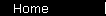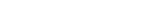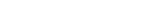# CAPM Calculator

Valuation with the Capital Asset Pricing Model uses a variation of discounted cash flows; only instead of giving yourself a "margin of safety" by being conservative in your earnings estimates, you use a varying discount rate that gets bigger to compensate for your investment's riskiness. There are different ways to measure risk; the original CAPM defined risk in terms of volatility, as measured by the investment's beta coefficient. The formula is:

Kc   =   Rf   +   beta x ( Km - Rf )
where
Kc is the risk-adjusted discount rate (also known as the Cost of Capital);
Rf is the rate of a "risk-free" investment, i.e. cash;
Km is the return rate of a market benchmark, like the S&P 500.

You can think of Kc as the expected return rate you would require before you would be interested in this particular investment at this particular price. The idea is that investors require higher levels of expected returns to compensate them for higher expected risk; the CAPM formula is a simple equation to express that idea.

Here is a calculator to let you try it out. You can find values for beta via the box below.

 Benchmark Return Rates
Return available on an appropriate market benchmark investment (like the S&P 500):   %
Return available on a risk-free investment (cash, or government bond):   %
 Risk Factor
Your investment's Beta (relative to the market benchmark above):

 Results
Carry result to DCF calculator...

 Find Beta   Ticker:
You now plug this value for the discount rate into the discounted cash flow calculator - ignoring the advice underneath it about using conservative estimates for earnings.

Analysts sometimes use a more complicated value for beta, that grows with a company's debt level. There is also lots of controversy about whether beta, which measures past volatility, is sufficient or even relevant in predicting future risk. William F. Sharpe, who invented CAPM, discusses these issues in an online interview.

One big caveat: the sophistication of the sliding discount rate makes this approach potentially dangerous. If you get creative enough with the discount rate and long-term growth expectations, you can come up with some wildly unrealistic valuations. So if you do use this approach, you should check your results by using discounted cash flows the traditional way, just to make sure you aren't fooling yourself.

(And one more caveat: CAPM is part of Modern Portfolio Theory, whose adherents would prefer not to value individual stocks using CAPM or any other method: MPT fans like index funds, not stocks.)

home  |  article  |  glossary  |  calculator  |  about us  |  booksValuation IntroA Little TheoryDCF CalculatorP/E RatioP/S RatioPEG RatioGraham FormulaDividend DiscountBuffett Formula (?)CAPM CalculatorBooks & LinksValuation FormulaStock Market PredictionsMeasuring Investment ReturnsStock Market CAGR